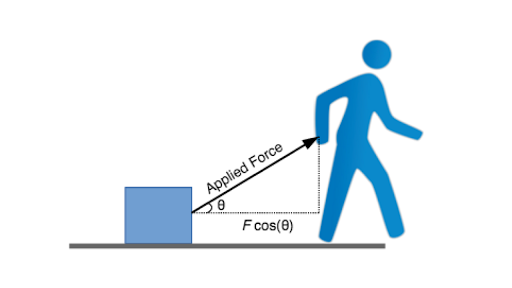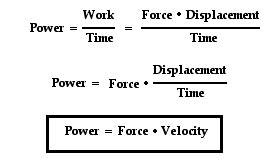# Define work and power.

Work, in physics, measure of energy transfer that occurs when an object is moved over a distance by an external force at least part of which is applied in the direction of the displacement.In physics, power is the rate of doing work, the amount of energy transferred per unit time. Having no direction, it is a scalar quantity. In the SI system, the unit of power is the joule per second (J/s), known as the watt in honour of James Watt, the eighteenth-century developer of the steam engine.• 2
Work is defined as the product of component of force in the direction of displacement and the magnitude of displacement.
Power is the rate of doing work.
• 0
What are you looking for?